# The physical limits of computation inspire an open problem that concerns abstract computable sets X⊆N and cannot be formalized in the set theory ZFC as it refers to our current knowledge on X

Kurpaska, Sławomir and Tyszka, Apoloniusz (2020) The physical limits of computation inspire an open problem that concerns abstract computable sets X⊆N and cannot be formalized in the set theory ZFC as it refers to our current knowledge on X. [Preprint]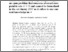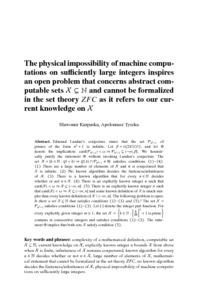Preview
Text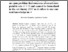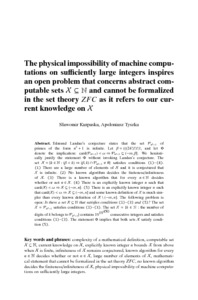Preview
Text
machine_computations.pdf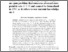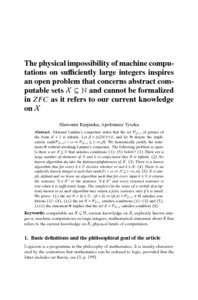Preview
Text
sk_at.pdf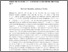Preview
Text
limits_of_computation.pdf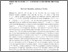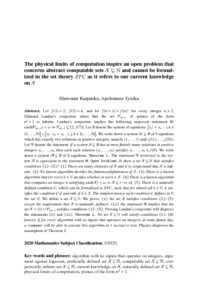Preview
Text
limits-of-computation.pdf

## Abstract

Let f(1)=2, f(2)=4, and let f(n+1)=f(n)! for every integer n≥2. Edmund Landau's conjecture states that the set P(n^2+1) of primes of the form n^2+1 is infinite. Landau's conjecture implies the following unproven statement Φ: card(P(n^2+1))<ω ⇒ P(n^2+1)⊆[2,f(7)]. Let B denote the system of equations {x_i!=x_k: i,k∈{1,...,9}}∪ {x_i⋅x_j=x_k: i,j,k∈{1,...,9}}. We write down a system U⊆B of 9 equations which has exactly two solutions in positive integers, namely (1,...,1) and (f(1),...,f(9)). Let Ψ denote the statement: if a system S⊆B has at most finitely many solutions in positive integers x_1,...,x_9, then each such solution (x_1,...,x_9) satisfies x_1,...,x_9≤f(9). We write down a system A⊆B of 8 equations. Theorem 1. The statement Ψ restricted to the system A is equivalent to the statement Φ. Open Problem: Is there a set X⊆N that satisfies conditions (1)-(5) ? *** (1) There are many elements of X and it is conjectured that X is infinite. (2) No known algorithm decides the finiteness/infiniteness of X . (3) There is a known algorithm that for every k∈N decides whether or not k∈X. (4) There is a known algorithm that computes an integer n satisfying card(X)<ω ⇒ X⊆(-∞,n]. (5) There is a naturally defined condition C, which can be formalized in ZFC, such that for almost all k∈N, k satisfies the condition C if and only if k∈X. The simplest known such condition C defines in N the set X. *** We define a set X⊆N. We prove: (i) the set X satisfies conditions (1)-(5) except the requirement that X is naturally defined; (ii) the statement Φ implies that the set X={1}∪P(n^2+1) satisfies conditions (1)-(5). Proving Landau's conjecture will disprove the statements (i) and (ii). Theorem 2. No set X⊆N will satisfy conditions (1)-(4) forever, if for every algorithm with no inputs that operates on integers, at some future day, a computer will be able to execute this algorithm in 1 second or less. Physics disproves the assumption of Theorem 2.

 Export/Citation: EndNote | BibTeX | Dublin Core | ASCII/Text Citation (Chicago) | HTML Citation | OpenURL
 Social Networking:

Item Type: Preprint
Creators:
CreatorsEmailORCID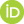Tyszka, Apoloniuszrttyszka@cyf-kr.edu.pl0000-0002-2770-5495
Keywords: algorithm with no inputs that operates on integers; argument against logicism; artificially defined set X⊆N; computable set X⊆N; conjecturally infinite set X⊆N; current knowledge on X; naturally defined set X⊆N; physical limits of computation; primes of the form n^2+1
Subjects: Specific Sciences > Mathematics > Foundations
Specific Sciences > Mathematics > Logic
Depositing User: Apoloniusz Tyszka
Date Deposited: 09 Oct 2020 17:41
Item ID: 18200
Subjects: Specific Sciences > Mathematics > Foundations
Specific Sciences > Mathematics > Logic
Date: 16 June 2020
URI: http://philsci-archive.pitt.edu/id/eprint/18200

### Monthly Views for the past 3 yearsView Item